7.5. FOUR-BAR AND SLIDER-CRANK COGNATES ROBERTS - CHEBYCHEV THEOREM

When our concern is only the path traced by the coupler point of a four-bar or a slider-crank mechanism, we can determine other four-bar or slider-crank mechanism proportions that generate identically the same coupler point curve. The four-bar mechanisms that generate the identical coupler point curve are known as the cognates of a four-bar. Let us state a theorem and show how the cognates of a four-bar and slider crank mechanism can be found.

Roberts-Chebychev Theorem

There are three different four-bar mechanism proportions that will trace identically the same coupler curve.

Consider the four-bar mechanism shown (A0B0=a1, A0A=a2, AB=a3, B0B=a4,  AP=b3, BP=c3).  The coupler point P traces a curve as shown. For this mechanism the loop equation is:(1)

The location of coupler point, P, for any crank angle q12 can be determined from the equations: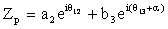(2)

or(3)

Noting that the angles q13, q14, are determined for every crank angle q12 from the loop equation.Figure  7.33.

The construction for determining the cognates can be given by the following steps (Fig.7.34):

1. Draw A0A1//AP and B0B1//BP

2. Draw PA1//A0A and  PB1//B0B

Hence A0A1PA and B0B1PB form parallelograms. Therefore:

A0A1=b3, A1P=a2, B0B1=c3, B1P=a4

Also:

< xA0A1=q12+a,  < xA1P=q12,  < xB0B1=q13-b, < xB1P=q14

• Construct triangles DPA1B1 and DPB1C2 similar to the triangle DPAB
( < C2B1P=< PBA;    < PA1C1 =< BAP);

From the similarity of the triangles:or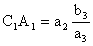orand similarly:,Also < xA1C1=q12+a,  < xB1C2= q13+p - b,

< xC2P=q12+a ,   < xPC1=q13+p - b.

4.   Draw   C1C0 //PC2 and C2C0// C1P  to locate C0. C0C1PC2 forms a parallelogram   therefore: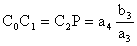and  < xC1C1=q12+a,           < xC0C2= q13+p - b

5. Four bar mechanisms that trace the same coupler curve are:

A0ABB0    (P on AB)  (a)

C0C1A1A0  (P on C1A1)  (b)C0C2B1B0   (P on C2B1 )  ( c)

Now, the location of C 0 can be written as :

A0C0=A0A1+A1C1+C1C0

or

A0C0=A0B0+B0B1+B1C2+C2C0

Writing these equations in complex numbers:

 A0C0=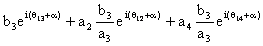(4)

or

 A0C0=(5)

Equations (4) and (5) can also be written as:

 A0C0=(6)
 A0C0=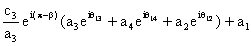(7)Figure  7.36

From equation (1) the terms inside the parenthesis are equal to a1. Therefore, all the three four-bar mechanisms

satisfy the same loop equation and point C0 is a fixed point located at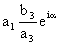.The angles q13, q14 and q12

are all related by equation (1) for all the three four-bar mechanisms and the coordinates of the coupler point P on the coupler point of all these three  four-bar mechanisms are given by equation (2) or (3). Hence the theorem is proved.

The given procedure locates both the orientation and the lengths of the links for all the four-bar cognates. A simpler construction for determining the link lengths is to stretch the four-bar mechanisms such that A0ABB0 lie on a straight line, since the theorem is also valid for an immovable four-bar with a new fixed link length a’1=a2+a3+a4. Application of the above steps to such a configuration for determining its cognates is quite simple as shown in the figure. One can then determine the location C0 of the original configuration by noting

 A0C0 ==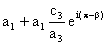,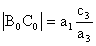One can join any two or all three of the cognates by a revolute joint at P and the mechanism thus obtained will still be movable (provided that the cognates are movable). The mechanism will be of permanent critical form (the general degree-of-freedom equation will not apply).

Another important use of the cognates of four-bar mechanisms is to obtain a rigid body motion which is in a translation (e.g. lines taken on this body remain parallel to their original positions) and every point on this body describe paths which are the same as the path of coupler point of the original four-bar.

Referring to figures,  note that the cranks A0A and C0C2 or B0B and C0C1 or B0B1 and A0A1  rotate by the same amount although these angles differ by a constant (a or p-b).  Let us move one of the cognates relative to the other such that the centers of rotation of the two cranks rotating at the same speed are coincident.  For example, if  C0 and A0 are to be made coincident every point of the cognate C0C2B1B0  must be displaced parallel to A0C0 by an amount |A0C0| . The relative positions of all the links of the cognate with respect to each other are kept, e.g. since the cranks C0C2 and A0A rotate at the same speed, their relative positions will not change and hence they can be connected to each other. Now consider points P and P’. Both of these points will always describe the same path and the distance PP’ will not change and  will always be parallel to the initial line drawn. Therefore, we can attach a link in between the two points PP’ and connect them by revolute joints to the coupler links of the two four-bars. The seven link mechanism thus obtained is an overclosed mechanism with link PP’ in a translation. We can eliminate this overclosure by removing one of the cranks B0B or C0C2  and obtain a six-link mechanism as shown. The mechanism can still perform a constrained motion with PP’ in translation. Note that the six link mechanism for the required motion is not unique (there are other six-link mechanisms to generate a translation of a link as that of point P.

Example 4.9.

Mechanism shown below is known as Chebyshev lambda or Hoecken straight line mechanism. The coupler point P describes an approximate straight-line. The link length proportions are given as: AB = BP = B0B = 2.5 A0A,  A0B0 = 2 A0A.  We would like to obtain a rigid body in an approximate rectilinear translation guided by a six-link mechanism.Figure  7.39(a)

The construction of the cognates and the design of the six link mechanism is shown in the following flash fileResulting mechanism

This mechanism has been used as the suspension system for an off-road vehicle.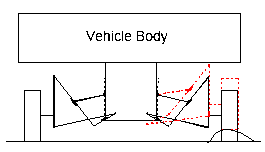In case of slider-crank mechanisms, there are two different slider-crank proportions that trace the same coupler-point curve. The construction for determining the cognate of a slider-crank mechanism can be outlined by the following steps (Proof is left as an exercise).
1. Draw PA1//AA0and A0A1//PA
2. Draw triangle DPA1C1  similar to the triangle DPAB (< A1PC1 = < ABP )One can also extend A0AB on a straight line and obtain the link length dimensions as shown..If the designer's main concern is simply the coupler-point curve, then he can easily replace a four-bar or slider-crank mechanism with its cognates. The cognate may have a better dimensional characteristics.